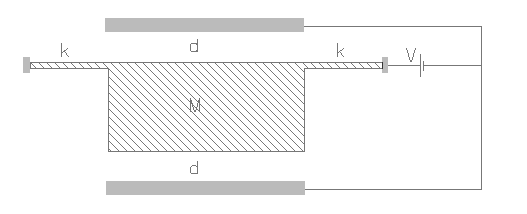ACLPULSE,k,area,m,gap,volt,g,np,rper,sel
Capacitive accelerometer for sensing a pulse signal

k            spring constant of the beam in N/m
area      overlapping area of the electrodes in µm2
m           inertial mass in kg
gap        distance between the stationary and movable plate in µm
volt        applied voltage in Volt
g            amplitude of the pulse signal in g
np          number of active stationary plates, 1 plate by default
rper       relative permittivity of the medium, it is approximately 1 for air
sel         number denoting the selected result.
Use 2 for maximum time duration of pulseNotes

This interface could be used for designing a capacitive accelerometer meant to sense or operate under a pulse signal. Pulse signal has a large amplitude of acceleration acting for a very short period of time. Pulse signals are usually the worst form of shock loads that the accelerometer will face during operation and hence should be designed to survive this kind of load. For any given magnitude of acceleration a corresponding maximum value for the pulse duration can be found out.

The accelerometer has a mass suspended from a beam with a known spring constant. The stationary electrode can be one or two as shown in the figure above. For capacitance measurement an electrical excitation is applied between the stationary and movable electrodes. This electrical excitation also exerts an electrostatic attraction on the mass which has the movable electrode. The combination of the electrostatic force and the inertial force should not be large enough to send the mass into a pulled-in state where the mass hits one of the stationary electrodes. For single sided excitation, the mass has an offset from the equilibrium position due to the electrical excitation. For double sided excitation, this effect is cancelled off.

The capacitor design or the applied voltage can be changed to increase the range of operation. The applied voltage usually has a AC component and a DC component typically in the form of ±V1±V2sinωt where frequency ω is much larger than the frequency of the signal and the natural frequency of vibration of the accelerometer. The effective applied voltage can be expressed as

V = √(V12 + V22∕2)

The plot shows acceleration measured in terms of acceleration due to gravity g versus the maximum pulse time in µsec. The plot shows that the allowable pulse duration drops off rapidly with the magnitude of acceleration.  A pulse duration above this maximum value can make the system unstable.  It can also be seen that for double sided excitation, the operational range is larger than for single sided excitation. For the same accelerometer and supply voltage, the maximum allowable acceleration or pulse duration is slightly higher for double sided excitation.

Assumptions

-Pulse acceleration signal normal to the plane of the mass is assumed.
-Damping effects are not considered.
-The pulse duration is small and the magnitude of acceleration is large
-The stationary electrodes are arranged symmetrically on both sides of the movable electrode.
-The spring constant of the beam is known. If unknown, this can be calculated under Mechanics > Structures > Beams.
-The medium between the electrodes is air by default with a relative permittivity of 1.
-The gap between the electrodes is uniform.
-The supply voltage is constant.
-The static displacement of the mass before the application of voltage and acceleration signal is considered to be zero.